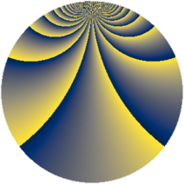# Properties

 Label 3630.2.btLevel $3630$ Weight $2$ Character orbit 3630.bt Rep. character $\chi_{3630}(47,\cdot)$ Character field $\Q(\zeta_{220})$ Dimension $21120$ Sturm bound $1584$

# Related objects

## Defining parameters

 Level: $$N$$ $$=$$ $$3630 = 2 \cdot 3 \cdot 5 \cdot 11^{2}$$ Weight: $$k$$ $$=$$ $$2$$ Character orbit: $$[\chi]$$ $$=$$ 3630.bt (of order $$220$$ and degree $$80$$) Character conductor: $$\operatorname{cond}(\chi)$$ $$=$$ $$1815$$ Character field: $$\Q(\zeta_{220})$$ Sturm bound: $$1584$$

## Dimensions

The following table gives the dimensions of various subspaces of $$M_{2}(3630, [\chi])$$.

Total New Old
Modular forms 64000 21120 42880
Cusp forms 62720 21120 41600
Eisenstein series 1280 0 1280

## Trace form

 $$21120q - 8q^{7} + O(q^{10})$$ $$21120q - 8q^{7} - 36q^{10} - 72q^{13} - 528q^{16} + 16q^{21} + 84q^{22} - 24q^{25} + 12q^{28} + 16q^{31} + 92q^{33} - 16q^{36} + 16q^{37} + 32q^{42} + 128q^{43} - 32q^{45} + 24q^{46} + 88q^{51} + 24q^{52} - 20q^{55} + 48q^{57} + 84q^{58} + 48q^{61} - 180q^{63} + 8q^{66} + 48q^{67} + 84q^{70} - 76q^{73} - 96q^{75} + 16q^{78} + 56q^{81} + 16q^{85} - 64q^{87} - 4q^{88} - 48q^{90} + 768q^{91} - 28q^{93} - 40q^{97} + O(q^{100})$$

## Decomposition of $$S_{2}^{\mathrm{new}}(3630, [\chi])$$ into newform subspaces

The newforms in this space have not yet been added to the LMFDB.

## Decomposition of $$S_{2}^{\mathrm{old}}(3630, [\chi])$$ into lower level spaces

$$S_{2}^{\mathrm{old}}(3630, [\chi]) \cong$$ $$S_{2}^{\mathrm{new}}(1815, [\chi])$$$$^{\oplus 2}$$